Right Square & Right Rhombic Pyramid

EQUABLE RIGHT SQUARE & RIGHT RHOMBIC PYRAMID

Balmoral Software

 Solutions: 10 (square) Solutions: ∞ (rhombic)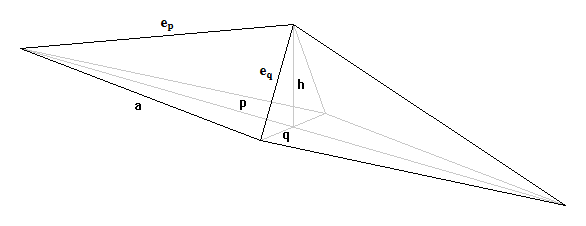There are exactly 10 equable right square pyramids and infinitely-many equable right rhombic pyramids. Denote the area of the rhombus base by the positive integer B, the area of one of its four congruent lateral triangular faces by the positive integer T, and its volume by the integer V. Since each triangular face is a vertical projection above one-quarter of the base, it is evident that
 T > B/4 1 ≤ B ≤ 4T - 1 
If h represents the height of the pyramid, then
V = (1/3)Bh
By equability, the total surface area is
 S = B + 4T = V, 
so the height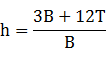is rational. The relationship between the side a and the diagonals p and q of the rhombus base is
 p2 + q2 = 4a2 
The area of the rhombus can be expressed in terms of its diagonals as
 B = pq/2 
Since every rhombus is orthodiagonal, each triangular face of the pyramid is the base of a trirectangular tetrahedron having edge lengths p/2, q/2 and h bounding the trihedral angle. The square of the area of the triangular face then is
 T2 = (1/4)[(p/2)2h2 + (q/2)2h2 + (p/2)2(q/2)2)] 
Substituting  and  into , we have
T2 = a2h2/4 + B2/16
Using , this reduces to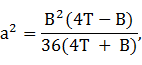and so a2 is rational. Collecting terms in T, we have
4(B2 - 36a2)T = B3 + 36a2B > 0
Therefore, an equable solution must have
B > 6a
Since a non-square rhombus is non-cyclic, its area does not exceed that of the corresponding square:
 B ≤ a2, 
from which it follows that
 B ≥ 37 
Substituting  in ,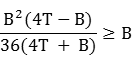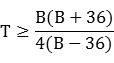For B ≥ 37, the right side of  is minimized at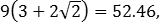so T must be at least 53. The inequality in  can also be written
 B2 - (4T - 36)B + 144T ≤ 0 
Without loss of generality, we can take p ≥ q and write  as
 q = 2B/p 
Substituting into , we have
p2 + (2B/p)2 = 4a2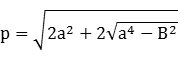The lateral edge lengths of the pyramid are the hypotenuses associated with the mutually-orthogonal half-diagonals p/2 and q/2 and the height h: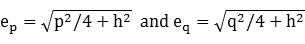Since T is unbounded, there are infinitely-many equable right rhombic pyramids. They can be generated over the following domain:
• T ≥ 53
• 37 ≤ B ≤ 4T - 1 by  and 
• If inequality  holds:
• Calculate V by , h by  and a2 by 
• Calculate p and q by  and 
• Calculate ep and eq by 
For example, when T = 53, there are 21 equable right rhombic pyramids:
BThV=Sapqepeqp/q
7853145/13 = 11.15382908.836812.793012.194212.857812.71151.05
7953873/79 = 11.05062918.901313.062612.095612.836412.59731.08
8053219/20 = 10.95002928.964713.267912.059112.802812.50031.10
8153293/27 = 10.85192939.026813.442512.051412.764712.41261.12
8253441/41 = 10.75612949.087813.596612.061912.724412.33151.13
8353885/83 = 10.66272959.147713.735012.085912.682912.25601.14
8453 74/7 = 10.57142969.206313.860612.120712.640612.18541.14
8553891/85 = 10.48242979.263813.974712.164912.597712.11921.15
8653447/43 = 10.39532989.320214.078312.217412.554412.05731.15
8753299/29 = 10.31032999.375314.172112.277712.510611.99951.15
8853225/22 = 10.22733009.429314.256112.345612.466211.94571.15
8953903/89 = 10.14613019.482214.330612.421012.421111.89591.15
9053151/15 = 10.06673029.533814.395212.504112.375111.85021.15
9153909/91 = 9.98903039.584314.449512.595612.327911.80861.15
9253228/23 = 9.91303049.633614.492712.696012.279211.77141.14
9353305/31 = 9.83873059.681814.523612.806812.228411.73901.13
9453459/47 = 9.76603069.728714.540212.929612.174911.71191.12
9553921/95 = 9.69473079.774514.539613.067812.117711.69101.11
9653 77/8 = 9.62503089.819114.516013.226712.054911.67811.10
9753927/97 = 9.55673099.862614.458013.418211.982911.67661.08
9853465/49 = 9.48983109.904814.328413.679111.890411.69771.05
For T ≤ 1000, there are 1,889,244 equable right rhombic pyramids.

All five pyramid faces have the same area if and only if h = 15, which is equivalent to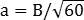.
Also, the base side a is an integer if and only if h = 8, which is equivalent to a = B/12.

Proof. For the first part, note that in ,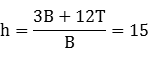is equivalent to T = B. Then from ,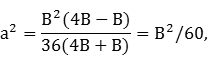so.

For the second part, note that  is equivalent to

 h = 3 + 12T/B 
and therefore,
 4T = B(h/3 - 1) 
If h = 8 in , then 12T/B = 5, or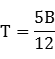Since T ∈ ℤ and 12 is relatively prime to 5, it follows that 12 | B, or B/12 ∈ ℤ. Substituting  in ,
a2 = (B/12)2,
so a ∈ ℤ.

Conversely, note that by substituting  in , we haveIf a ∈ ℤ, then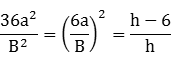is the square of a rational, so we can write
h - 6 = mx2
and
h = my2
for some positive integers m, x, y. It follows that
my2 - mx2 = 6

m(y2 - x2) = 6

Since the left side is a product of two integers, we have the following possibilities:
 m y2 - x2 1 6 Difference of two squares cannot be 6 2 3 Only possibility is 22 - 12 3 2 Difference of two squares cannot be 2 6 1 Difference of two squares cannot be 1
The only solution is h = my2 = (2)22 = 8 and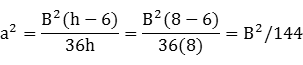so a = B/12, QED.

### Right Square Pyramids

For a square base, there is equality in :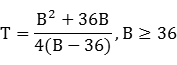Since T is an integer, B - 36 must divide the numerator. The integer quotient is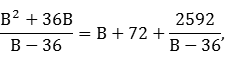so B - 36 must divide 2592. Thirty candidate values for B can then be generated from the divisors of 2592. Of these, ten produce integer values of T in . The remaining parameters can all be determined in terms of B:
From , h = 6B/(B - 36)

From , V = S = 2B2/(B - 36)

From , a2 = B

a4 - B2 = 0

p2 = 2a2 = 2B by 

p2/4 = B/2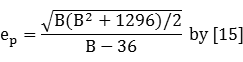The equable right square pyramids are listed below, ordered by volume:
Base area BTriangle face area THeight hV = SBase side aLateral edge
7254122888.485313.4164
6060153007.746015.9687
10854932410.392311.6189
4884243846.928224.4949
1446083841211.6619
44110334846.633233.3317
25284758815.874513.2288
40190608006.324660.1664
36011020/380018.973714.9815
68419019/3144426.153419.5477
The only solution with the same area for all five pyramid faces is B = T = 60, h = 15 and V = S = 300.
The only solution with an integer base side is a = 12, h = 8 and V = S = 384.